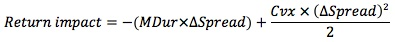### Why should I choose AnalystNotes?

AnalystNotes specializes in helping candidates pass. Period.

##### Subject 5. Credit Risk vs. Return: Yields and Spreads
The higher the credit risk, the greater the required yield and potential return demanded by investors. Over time, bonds with more credit risk offer higher returns but with greater volatility of return than bonds with lower credit risk.

Yield spread is the difference in yield between two securities.

• The yield of a corporate bond = yield on a risk-free bond + yield spread

Yield spreads, especially credit spreads, become wider during economic contractions. In times of credit improvement or stability, however, credit spreads can narrow sharply as well. This is known as "flight to quality".

Factors that affect yield spreads include: the credit cycle, economic conditions, financial market performance, market making capacity, and supply/demand conditions.

How do spread changes affect the price of and return on these bonds? The impact depends on two factors:

• The basis point spread change
• The sensitivity of price to yield as reflected by modified duration and convexityA credit curve is essentially the spread over treasuries of various maturities for a single bond issuer. It is typically upward-sloping, meaning the longer the bond maturity, the wider the spread.

Learning Outcome Statements

i. describe factors that influence the level and volatility of yield spreads;

CFA® 2021 Level I Curriculum, , Volume 5, Reading 47

User Comment
BrettGardner10 Know return impact formula
Shaan23 Im letting this formula slide
ldfrench yuppppp^
robbiecow This is the same formula we have been looking at. The best way to remember this formula is thinking of Taylor Series.

(-1)*dP/dy + d^2P/dy^2
P = Price
f'(P) = duration
f''(P) = convexity
Memeteau thks robbie. I missed that f''(P) was convexity. I miss much stuff about maths. ;-)
sshetty2 nice one